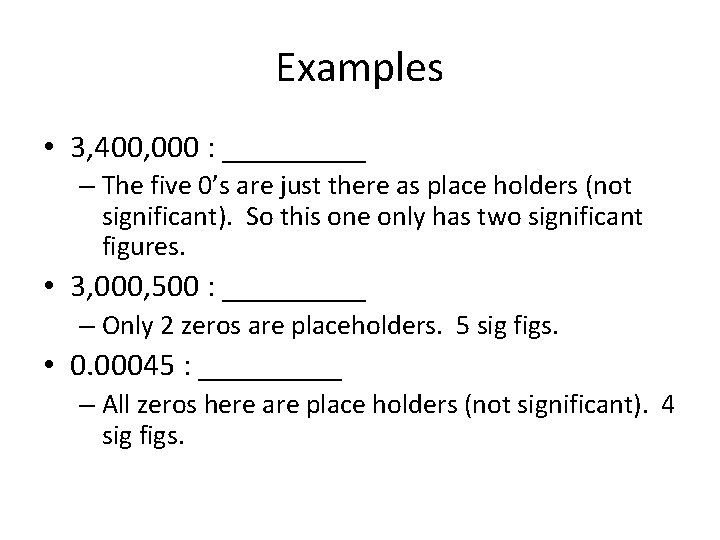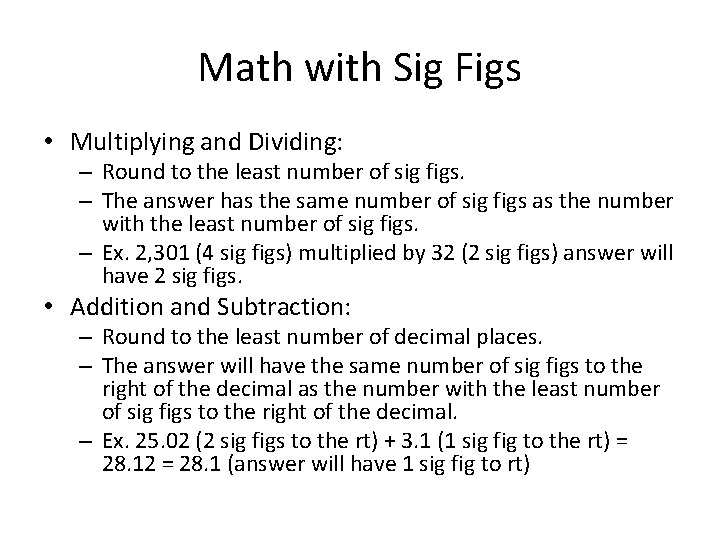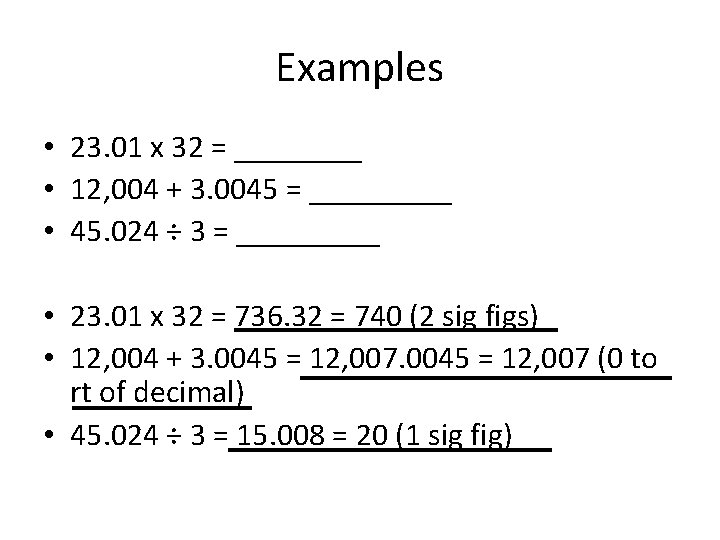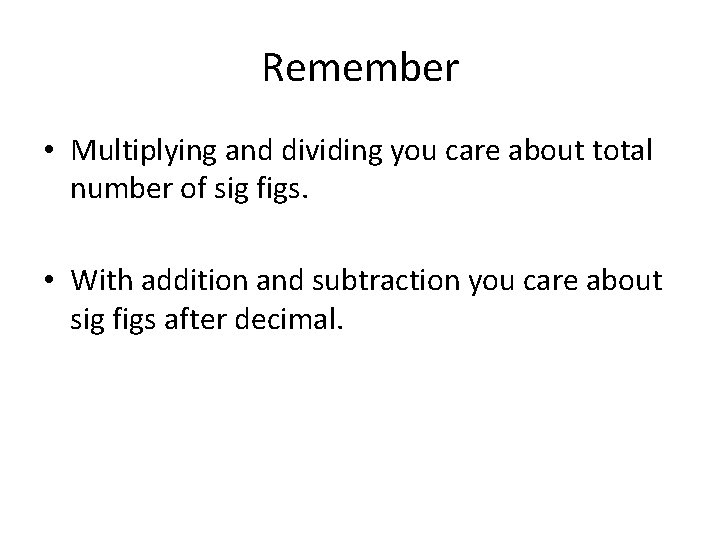# Scientific Notation and Significant Figures Scientific Notation Purpose

• Slides: 10Scientific Notation and Significant FiguresScientific Notation • Purpose: – To simplify writing of large or small numbers (faster) – To show significant figures (more obvious later)How to Write • 1 digit, then the decimal, then any other significant digits, then x 10 x • 3, 400, 000 = 3. 4 x 106 • Remember: – Positive exponents = a bigger number (you are adding zeros to the right hand side). – Negative exponents = a smaller number (less than 1) therefore you move the decimal to the left.Examples • 0. 003 = _______ – 3 x 10 -3 • 32, 000, 500 = _______ – 3. 20005 x 107 – Dropped the last 2 zeros because they are not significantSignificant Figures • All digits are significant that are not just there to show a big or little number (just place holders). • All non-zeros are significant. • Zeros in between non-zeros are significant. • All trailing zeros after a decimal are significant.Examples • 3, 400, 000 : _____ – The five 0’s are just there as place holders (not significant). So this one only has two significant figures. • 3, 000, 500 : _____ – Only 2 zeros are placeholders. 5 sig figs. • 0. 00045 : _____ – All zeros here are place holders (not significant). 4 sig figs.Harder Ones • 3, 400, 000. 0 : _____ • 0. 004200 : _____ • Now put in scientific notation: – 3. 400000 x 106 (scientific notation only shows significant figures). – 4. 200 x 10 -3Math with Sig Figs • Multiplying and Dividing: – Round to the least number of sig figs. – The answer has the same number of sig figs as the number with the least number of sig figs. – Ex. 2, 301 (4 sig figs) multiplied by 32 (2 sig figs) answer will have 2 sig figs. • Addition and Subtraction: – Round to the least number of decimal places. – The answer will have the same number of sig figs to the right of the decimal as the number with the least number of sig figs to the right of the decimal. – Ex. 25. 02 (2 sig figs to the rt) + 3. 1 (1 sig fig to the rt) = 28. 12 = 28. 1 (answer will have 1 sig fig to rt)Examples • 23. 01 x 32 = ____ • 12, 004 + 3. 0045 = _____ • 45. 024 ÷ 3 = _____ • 23. 01 x 32 = 736. 32 = 740 (2 sig figs) • 12, 004 + 3. 0045 = 12, 007 (0 to rt of decimal) • 45. 024 ÷ 3 = 15. 008 = 20 (1 sig fig)Remember • Multiplying and dividing you care about total number of sig figs. • With addition and subtraction you care about sig figs after decimal.